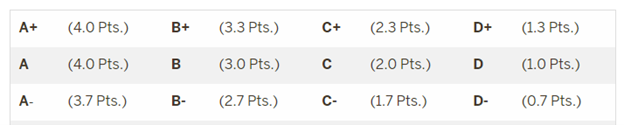# IUPUI Campus Bulletin 2022-2023

IUPUI Bulletins » Policies » Academic Policy » Grade Point Average (GPA)

In addition to completing all the required course work, students must have a specific overall grade point average (GPA) and a specific GPA in their major to graduate. Students should familiarize themselves with the policies of their academic program.

The following grades are considered in computing semester or cumulative grade averages.The following grades are not considered in computing semester or cumulative grade point averages:

P          Passed (Pass/Fail Option)
S          Satisfactory
R          Deferred
I           Incomplete
W         Withdrawn

Semester and Cumulative GPAs

Only courses with grades of A+ through D– and F are used in calculating grade point averages (GPA). P and S grades are passing grades in completed courses and will count towards hours earned but they are not used in the calculation of a GPA.  I and R grades have no GPA value.  Courses taken at other non–IU institutions are not used in calculating the GPA.  An exception applies to Purdue transfer students who enter into a Purdue-mission program at IUPUI.  This course work is displayed with course hours and grades and, in addition, this work will be included in the Student Program GPA statistics.

• GPA Hours: the sum of hours in courses with a grade of A+ through D- or F
• GPA Points: the sum of the credit points for each course with a grade of A+ through D- or F. Credit points are calculated by multiplying the point per credit hour by the hours of credit for the course.  The points per credit hour are awarded based on the grade earned in the course as described in the Grading System chart above.
• Hours Earned: the number of course hours completed with a passing grade (A+ through D-, S, P)
• GPA: calculated by dividing the total GPA points by the total number of credit hours

Multiple GPA statistics may appear on a student’s transcript:

• Semester GPA: sum of all grade points earned in a semester divided by the sum of credit hours with grades of A+ through F
• Cumulative GPA: sum of all grade points earned in all courses, all semesters taken at IUPUI (or an IU campus) divided by all the credit hours with grades of A+ through F
• Program Summary GPA: based on the student’s last active primary program, includes all grades and hours earned by level (undergraduate, graduate, law, etc.)
• Degree GPA: In some cases, schools calculate a degree GPA, which may vary slightly from the program summary GPA.  This value appears in the summary portion of a student’s transcript. This variation is due to differences in program requirements, especially when students are earning Purdue degrees.  The degree GPA for IUPUI students pursuing Purdue degrees offered by the Schools of Engineering and Technology or Science will be calculated using the Purdue University grading policy. Courses taken at any IU or Purdue campus and included in the student’s program of study will be used in calculating the final GPA at the time the degree is awarded.